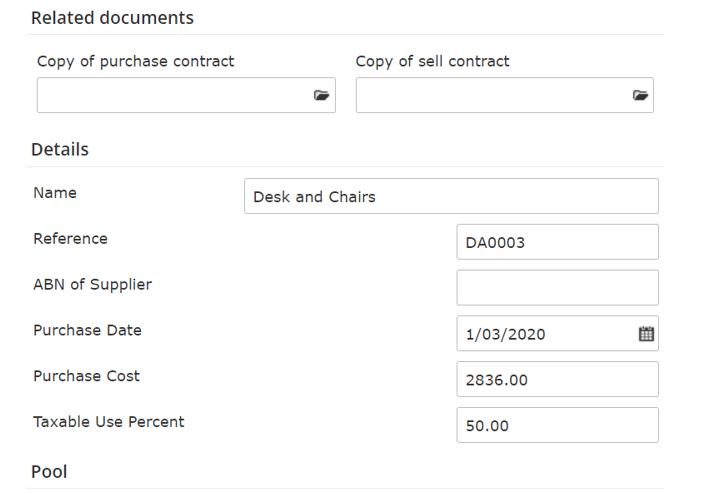Question: I need to calculate the depreciation on a low value pool based on a 50% taxable use.

I have added the low value pool and asset and 50% taxable use, however the calculation does not take into the 50% taxable use and is calculation the tax on 100%.Answer: The "Taxable Use Percent" is used only in income year when you add an asset to the pool to calculate the asset cost to be allocated. In this case it's 19-20 year. If you run the report for 19-20 you'll see how the taxable use % was applied. Thus, in 20-21 and future years the taxable use % is already included in the pool balance.